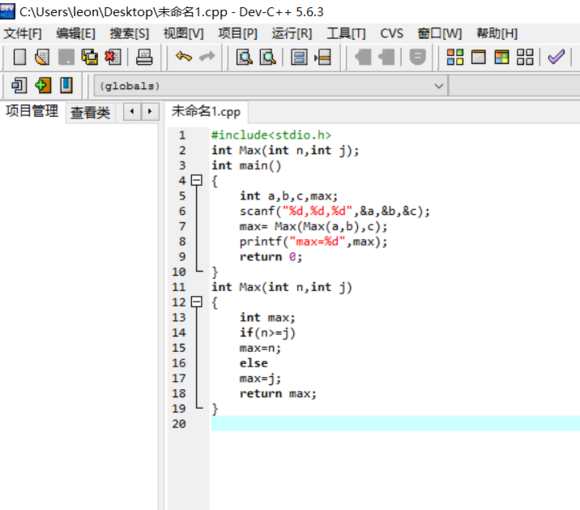2016-11-17 15:08

# dev 运行结果出错，求大神#include
int Max(int n,int j);
int main()
{
int a,b,c,max;
scanf("%d,%d,%d",&a,&b,&c);
max= Max(Max(a,b),c);
printf("max=%d",max);
return 0;
}
int Max(int n,int j)
{
int max;
if(n>=j)
max=n;
else
max=j;
return max;
}

• 点赞
• 写回答
• 关注问题
• 收藏
• 邀请回答